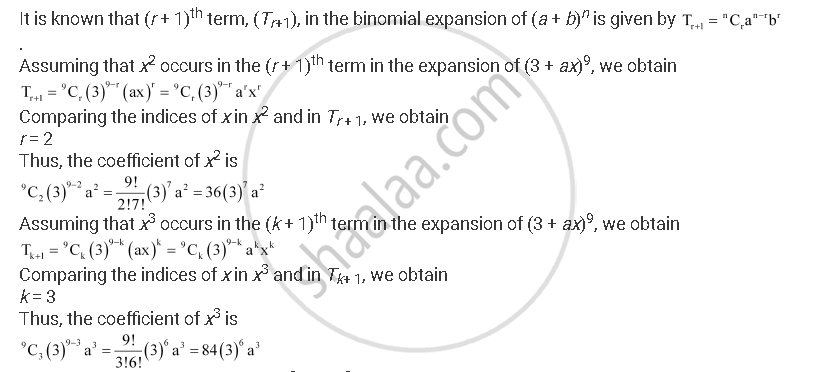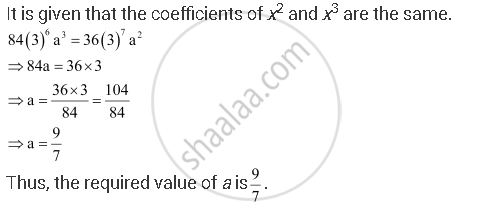CBSE (Arts) Class 11CBSE
Share

# Find a If the Coefficients of X2 and X3 in the Expansion of (3 + Ax)9 Are Equal. - CBSE (Arts) Class 11 - Mathematics

ConceptBinomial Theorem for Positive Integral Indices

#### Question

Find a if the coefficients of x2 and x3 in the expansion of (3 + ax)9 are equal.

#### SolutionIs there an error in this question or solution?

#### APPEARS IN

Solution Find a If the Coefficients of X2 and X3 in the Expansion of (3 + Ax)9 Are Equal. Concept: Binomial Theorem for Positive Integral Indices.
S